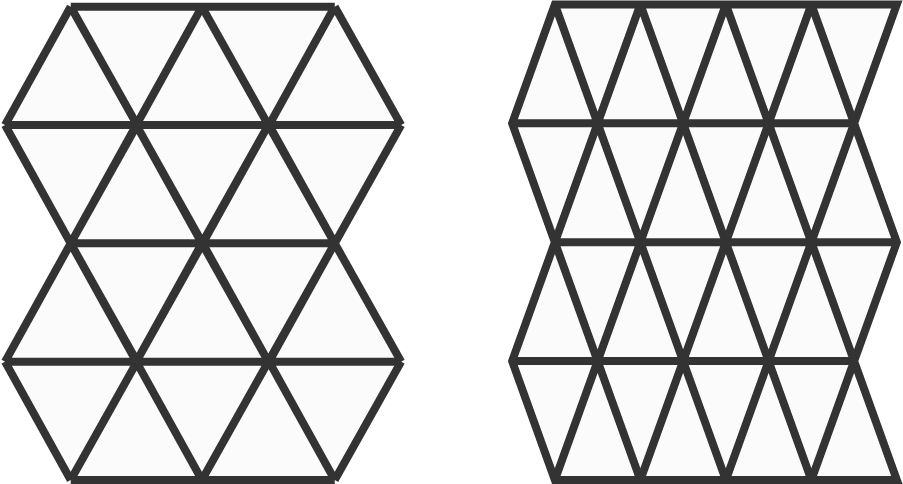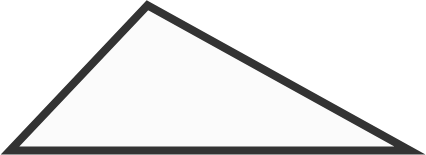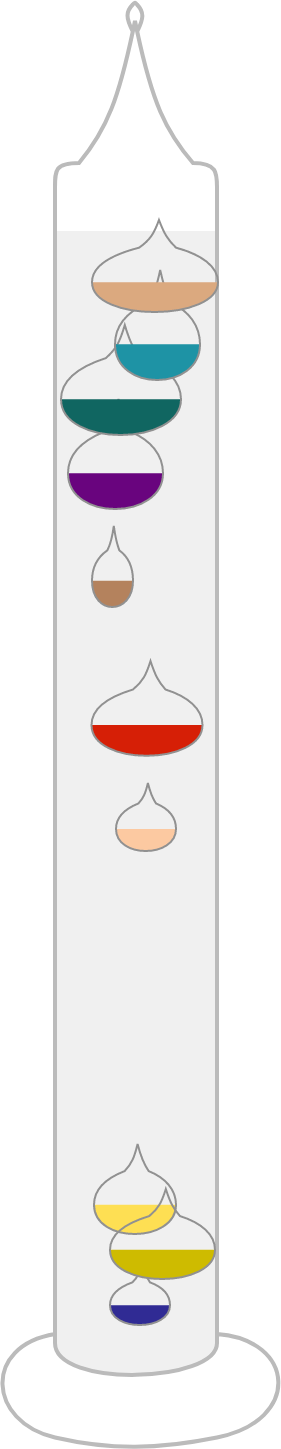# Problems of the Week

Contribute a problem

# 2018-06-18 Basic

An infinite plane can be tiled with identical equilateral or isosceles triangles with no overlaps and no gaps.Is it possible to tile an infinite plane with identical scalene triangles (identical triangles with distinct side lengths)?Note: Once you find a way to put the tiles together to cover a small area—as in the two examples shown—you can assume that the same method can cover an infinite plane.

If $x,x^2, x^3$ are three distinct real numbers, which of the following inequalities can be true?

Note: You can select more than one answer. This is a new answer format for Brilliant.

Select one or more

\begin{aligned} {\color{#D61F06}1}^5 &= {\color{#D61F06}1} \\ {\color{#D61F06}2}^5 &= 3{\color{#D61F06}2} \\ {\color{#D61F06}3}^5 &= 24{\color{#D61F06}3} \\ {\color{#D61F06}4}^5 &= 102{\color{#D61F06}4} \\ \end{aligned}

Is it true that the last digit of a whole number and that of its fifth power are always the same (in base 10)?The glass bulbs of a Galileo thermometer are sealed such that their densities are constant. The clear liquid in which the bulbs are submerged is ethanol, which changes density depending on the surrounding temperature.

Each bulb is labeled with the temperature at which its density is equal to that of the ethanol. For example, if the ethanol temperature is $20^\circ \text{C},$ then the bulb labeled $20^\circ \text{C}$ would have the same density as the ethanol.

At $25^\circ\text{C}$ ethanol temperature, what do you observe inside the thermometer?

Details and Assumptions:

• The density of ethanol decreases with increasing temperature.

$\begin{array}{ccccc} & & & A & B & C\\ - & & & & D & E \\ \hline & & & & & F \end{array}$

In the cryptogram above, the six letters are different digits and neither $A$ nor $D$ is zero.

Which of the following can be a value of $F?$

×